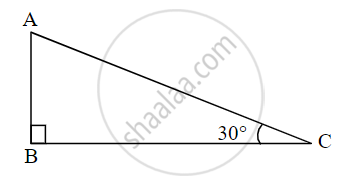# From given figure, In ∆ABC, If AC = 12 cm. then AB = ?Activity: From given figure, In ∆ABC, ∠ABC = 90°, ∠ACB = 30° ∴ ∠BAC = □ ∴ ∆ABC is 30° – 60° – 90 - Geometry

Sum

From given figure, In ∆ABC, If AC = 12 cm. then AB =?Activity: From given figure, In ∆ABC, ∠ABC = 90°, ∠ACB = 30°

∴ ∠BAC = square

∴ ∆ABC is 30° – 60° – 90° triangle

∴ In ∆ABC by property of 30° – 60° – 90° triangle.

∴ AB = 1/2 AC and square = sqrt(3)/2 AC

∴ square = 1/2 xx 12 and BC = sqrt(3)/2 xx 12

∴ square = 6 and BC = 6sqrt(3)

#### Solution

From given figure, In ∆ABC, ∠ABC = 90°, ∠ACB = 30°

∴ ∠BAC = 60°     ......[Remaining angle of a triangle]

∴ ∆ABC is 30° – 60° – 90° triangle.

∴ In ∆ABC,

by property of 30° – 60° – 90° triangle.

∴ AB = 1/2 AC    .......[Side opposite to 30°]

and BC = sqrt(3)/2 AC   .......[Side opposite to 60°]

∴ AB = 1/2 xx 12 and BC = sqrt(3)/2 xx 12

AB = 6 and BC = 6sqrt(3)

Concept: Right-angled Triangles and Pythagoras Property
Is there an error in this question or solution?

Share